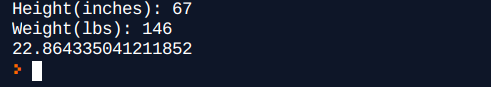Learn to Code via Tutorials on Repl.it

← Back to all posts
Calculating BMI for Adults in Python 3.0

# Calculating BMI for Adults in Python 3.0

@ChristianThone

## What is BMI?

BMI is Body Mass Index, it is a measurment of fat in a human body. By calculating your BMI you can figure out whether or not you are Underweight, Normal Weight, Overweight, or Obese.

## How do I find my BMI?

BMI is easy to find, first of you would need the formula, which is simple.

BMI = 703 * [Weight(lbs)] / [Height(Inches)]²

Using this formaula we can find BMI.

## Now getting to the code

Start of your code in the `main.py` file. Type the following code in:

``````import replit as sys
Height = ""
Weight = ""
BMI = ""
UserI = ""``````

As you can see we are importing `replit` as `sys` which will come in handy later. We are also defining a few variables.
`Height`: Height of the user that will be stored and used later.
`Weight`: Weight of the user that will be stored and used later.
`BMI`: BMI of the user which will be calculated later
`UserI`: User Input which will be used to record data from the user.

Now that you have those variables defined, its time to collect data from the user. Type in the following code to the `main.py` file:

``````Height = int(input("Height(inches): "))
Weight = int(input("Weight(lbs): "))``````

Here you can see that we used the variables: `Height` and `Weight`. If you look close you will see that `input()` is used this is pythons' way of collecting data from the user. If you look even closer you will see `int()` surrounding each `input()` this will convert user input into Integers.

Now for the actual math part of this, I will explain every part of the code so you can get a good idea about what is happening here.

In order to calculate BMI we need the formula which is shown at the top of this Tutorial.
Type the following code exactly as shown:

``BMI = 703 * Weight / Height ** 2``

Explanation:
So as you can see we used a few variables again: `BMI`, `Weight` and `Height`. The two variables `Height` and `Weight` store the previous input from the user. You can see the following Mathematical Operators in the formula: `/`, `*` and `**` I will explain each and their use.

`/`: Allows us to divide `Height` and 'Weight'
`*`: Allows us to multiply the Quotient of `Height` and `Weight` by 703 to convert to the metric system.
`**`: This is the basic math symbol telling the system that we want to have an exponent in the formula.

Now add `python print(str(BMI))` to the end of the code.
The `str` converts `BMI` to a string so it can print properly without error.
Now that we did that, your code should look something like this:

``````import replit as sys
Height = ""
Weight = ""
BMI = ""
UserI = ""

Height = int(input("Height(inches): "))
Weight = int(input("Weight(lbs): "))

BMI = 703 * Weight / Height ** 2

print(str(BMI))``````

Output:You can always fancy it up and make it look nice, have instructions, or whatever you would like to put into it. I have mine set up so it checks whether or not you are Underweight, Normalweight, Overweight, or Obese. You can view my project by clicking here